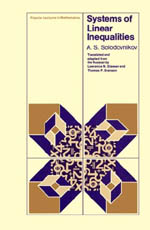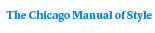# Systems of Linear Inequalities

A. S. Solodovnikov92 pages | 6.00 x 9.00 | © 1980
Paper \$26.00 ISBN: 9780226767864 Published February 1980
This volume describes the relationship between systems of linear inequalities and the geometry of convex polygons, examines solution sets for systems of linear inequalities in two and three unknowns (extension of the processes introduced to systems in any number of unknowns is quite simple), and examines questions of the consistency or inconsistency of such systems. Finally, it discusses the field of linear programming, one of the principal applications of the theory of systems of linear inequalities. A proof of the duality theorem of linear programming is presented in the last section.
Table of Contents• Contents
Contents
Foreword
1. Several Facts from Analytic Geometry
2. Geometric Interpretation of a System of Linear Inequalities in Two and Three Unknowns
3. The Convex Hull of a Point Set
4. Convex Polyhedral Cones
5. The Solution Domain of a System of Inequalities in Two Unknowns
6. The Solution Domain of a System in Three Unknowns
7. Systems of Linear Inequalities in an Arbitrary Number of Unknowns
8. Inconsistent Systems
9. Dual Convex Polyhedral Cones
10. The Duality Theorem of Linear Programming
References